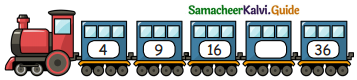Students can download 5th Maths Term 1 Chapter 3 Patterns Ex 3.2 Questions and Answers, Notes, Samacheer Kalvi 5th Maths Guide Pdf helps you to revise the complete Tamilnadu State Board New Syllabus, helps students complete homework assignments and to score high marks in board exams.

## Tamilnadu Samacheer Kalvi 5th Maths Solutions Term 1 Chapter 3 Patterns Ex 3.2

Question 1.
Square of the number 7 is
(a) 14
(b) 49
(c) 21
(d) 28
(b) 49
[72 = 7 × 7 = 49]Question 2.
64 is the square number of
(a) 4
(b) 16
(c) 8
(d) 32
(c) 8
[82 = 8 x 8 = 64]

Question 3.
Is 24 a square number?
NoQuestion 4.
A number multiplied by _____ is called square of that number.
itself

Question 5.
Fill in the blank box.